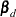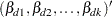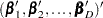### Logistic Regression Models

If the response categories of the response variablecan be restricted to a number of ordinal values, you can fit cumulative probabilities of the response categories with a cumulative logit model, a complementary log-log model, or a probit model. Details of cumulative logit models (or proportional odds models) can be found in McCullagh and Nelder (1989). If the response categories ofare nominal responses without natural ordering, you can fit the response probabilities with a generalized logit model. Formulation of the generalized logit models for nominal response variables can be found in Agresti (2002). For each model, the procedure estimates the model parameterby using a pseudo-log-likelihood function. The procedure obtains the pseudo-maximum likelihood estimator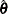by using iterations described in the section Iterative Algorithms for Model Fitting and estimates its variance described in the section Variance Estimation.

#### Cumulative Logit Model

A cumulative logit model uses the logit function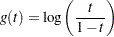Denote the cumulative sum of the expected proportions for the first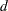categories of variableby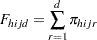for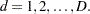Then the cumulative logit model can be written as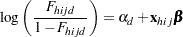with the model parameters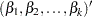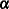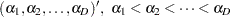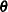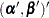#### Complementary Log-Log Model

A complementary log-log model uses the complementary log-log function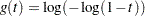as the link function. Denote the cumulative sum of the expected proportions for the firstcategories of variablebyforThen the complementary log-log model can be written as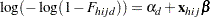with the model parameters#### Probit Model

A probit model uses the probit (or normit) function, which is the inverse of the cumulative standard normal distribution function,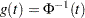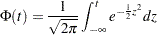Denote the cumulative sum of the expected proportions for the firstcategories of variablebyforThen the probit model can be written as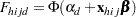with the model parameters#### Generalized Logit Model

For nominal response, a generalized logit model is to fit the ratio of the expected proportion for each response category over the expected proportion of a reference category with a logit link function.

Without loss of generality, let category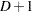be the reference category for the response variable. Denote the expected proportion for theth category by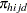as in the section Notation. Then the generalized logit model can be written as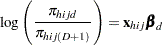for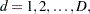with the model parameters